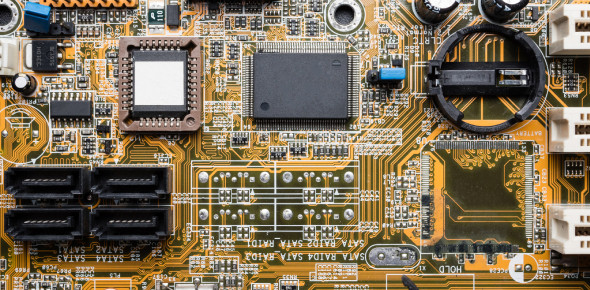# MCQ Quiz On Introduction To Semiconductors

20 Questions | Attempts: 2275
ShareSettingsPrepare yourself for this MCQ quiz on introduction to semiconductors. Semiconductors are meant by substances with properties somewhere between them. ICs(integrated circuits) as well as discrete electronic components, such as diodes and transistors, exist because of semiconductors. Common elemental semiconductors are known as silicon and germanium. Silicon is well-known of these. Silicon forms most of the integrated circuits. Let's see how much more you know! We wish you the best of luck!

• 1.
The term semiconductor arises from
• A.

Resistor-like properties of metal oxides.

• B.

Variable conductive properties of some materials.

• C.

The fact that electrons conduct better than holes.

• D.

Insulating properties of silicon and GaAs.

• 2.
Which of the following is not an advantage of semiconductor devices over vacuum tubes?
• A.

Smaller size

• B.

Lower working voltage

• C.

Lighter weight

• D.

Ability to withstand high voltage spikes

• 3.
Of the following substances, which is the most commonly used semiconductor?
• A.

Germanium

• B.

Galena

• C.

Silicon

• D.

Copper

• 4.
GaAs is
• A.

A compound

• B.

An element

• C.

A mixture

• D.

A gas

• 5.
A disadvantage of MOS devices is the fact that
• A.

The charge carriers move fast.

• B.

The material does not react to ionizing radiation.

• C.

They can be damaged by electrostatic discharges.

• D.

They must always be used at high frequencies.

• 6.
Selenium works especially well in
• A.

Photocells

• B.

High-frequency detectors

• C.

RF power amplifiers

• D.

Voltage regulators

• 7.
Of the following, which material allows the lowest forward voltage drop in a diode?
• A.

Selenium

• B.

Silicon

• C.

Copper

• D.

Germanium

• 8.
A CMOS integrated circuit
• A.

Can only work at low frequencies.

• B.

Requires very little power to function.

• C.

Requires considerable power to function.

• D.

Can only work at high frequencies.

• 9.
The purpose of doping is to
• A.

Make the charge carriers move faster.

• B.

Cause holes to flow.

• C.

Give a semiconductor material-specific properties.

• D.

Protect devices from damage in case of transients.

• 10.
A semiconductor material is made into N-type by
• A.

• B.

• C.

Injecting protons

• D.

Taking neutrons away

• 11.
Which of the following does not result from adding an acceptor impurity?
• A.

The material becomes P-type.

• B.

Current flows mainly in the form of holes.

• C.

Most of the carriers have a positive electric charge.

• D.

The substance acquires an electron surplus.

• 12.
In P-type material, electrons are
• A.

The majority carriers

• B.

The minority carriers

• C.

Positively charged

• D.

Entirely absent

• 13.
Holes move from
• A.

Minus to plus

• B.

Plus to minus

• C.

P-type to N-type material

• D.

N-type to P-type material

• 14.
When a P-N junction does not conduct even though a voltage is applied, the junction is
• A.

Reverse-biased at a voltage less than the avalanche voltage

• B.

Overdriven

• C.

Biased past the breaker voltage

• D.

In a state of the avalanche effect

• 15.
Holes flow the opposite way from electrons because
• A.

Charge carriers flow continuously.

• B.

They have an opposite electric charge.

• C.

They have the same electric charge.

• D.

Forget it! Holes flow in the same direction as electrons.

• 16.
If an electron is considered to have a charge of −1 unit, then a hole can be considered to have
• A.

A charge of −1 unit

• B.

No charge

• C.

A charge of +1 unit

• D.

A charge that depends on the semiconductor type

• 17.
When a P-N junction is forward-biased, conduction will not occur unless
• A.

The applied voltage exceeds the forward break-over voltage.

• B.

The applied voltage is less than the forward break-over voltage.

• C.

The junction capacitance is high enough.

• D.

The depletion region is wide enough.

• 18.
If the reverse bias exceeds the avalanche voltage in a P-N junction,
• A.

The junction will be destroyed.

• B.

The junction will insulate; no current will flow.

• C.

The junction will conduct current.

• D.

The capacitance will become extremely low.

• 19.
Avalanche voltage is routinely exceeded when a P-N junction acts as a
• A.

Current rectifier.

• B.

Variable resistor.

• C.

Variable capacitor.

• D.

Voltage regulator.

• 20.
Which of the following does not affect the junction capacitance of a diode?
• A.

The cross-sectional area of the P-N junction

• B.

The width of the depletion region

• C.

The phase of an applied ac signal

• D.

The reverse-bias voltage

## Related TopicsBack to top
×

Wait!
Here's an interesting quiz for you.